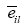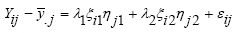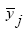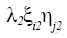# Abstracts

The objective of this study was to evaluate the use of SREG GGE biplot methodology and factor analysis to stratify the genotype×environment interaction in maize. Forty-nine early maize hybrids were evaluated in nine environments. The experimental design used was a 7×7 square lattice with two replicates. Each plot consisted of two 5m long rows spaced 0.90m apart. Grain yield data were used to perform the analysis. The results indicated the existence of two mega-environments in the State of Minas Gerais, Brazil, for early maize hybrids. The stratification of the environment by factor analysis was more selective to join the similarity the according with cultivar performance. However, this approach did not identify specific genotype x environment interactions, which is possible through SREG GGE biplot analysis.

PAPERS

CROP PRODUCTION

Factor analysis and SREG GGE biplot for the genotype × environment interaction stratification in maize

Análise de fatores e SREG GGE biplot para a estratificação da interação genótipos × ambientes em milho

Roberto Fritsche-NetoI; Glauco Vieira MirandaI,1 1 Autor para correspondência. ; Rodrigo Oliveira DeLimaI; Heraldo Namorato de SouzaI

IDepartamento de Fitotecnia, Universidade Federal de Viçosa (UFV), Av. PH Rolfs, s/n, Campus Universitário, 36570-000, Viçosa, MG, Brasil. E-mail: glaucovmiranda@ufv.br

ABSTRACT

The objective of this study was to evaluate the use of SREG GGE biplot methodology and factor analysis to stratify the genotype×environment interaction in maize. Forty-nine early maize hybrids were evaluated in nine environments. The experimental design used was a 7×7 square lattice with two replicates. Each plot consisted of two 5m long rows spaced 0.90m apart. Grain yield data were used to perform the analysis. The results indicated the existence of two mega-environments in the State of Minas Gerais, Brazil, for early maize hybrids. The stratification of the environment by factor analysis was more selective to join the similarity the according with cultivar performance. However, this approach did not identify specific genotype x environment interactions, which is possible through SREG GGE biplot analysis.

Key words:Zea mays L., adaptability, stability.

RESUMO

INTRODUCTION

The evaluation of experimental hybrids is the most difficult phase in a maize breeding program and requires much resource, because a large number of genotypes need to be evaluated in different environments (SOUZA et al., 2009). Therefore it would be interesting to determine the minimum number of that required evaluating the genotypes without reducing data quality and consequently to reduce the experimental costs (MENDONÇA et al., 2007).

Despite the importance of studying their adaptability and stability, in general, the recommendation of cultivars is based solely on the average performance in test environments. However, taking into account the responses of genotypes in different environments (genotype×environment interaction) may significantly increase the productive potential of the culture (MIRANDA et al., 2008).

There are several methods for evaluating the performance of hybrids and their genotypic interactions with the environment. They differ in the parameters used in the assessment, the biometric procedures employed, and the analysis (CRUZ & REGAZZI, 2001). The analysis of factors reported by MURAKAMI et al. (2004) is a multivariate technique that reduces the original number of variables observed to a small number of abstract variables called factors. These may be independent or correlated, and each factor combines those original variables that are strongly correlated with each other, but weakly correlated with other factors (JOHNSON & WICHERN, 1992). Thus, analysis of the factors may establish sub-environments with high correlations between performance within subgroups of hybrids and low or zero correlation between subgroups.

The analysis of a GGE (genotype and genotype-environment interaction) biplot was proposed for a graphic interpreting the genotype x environment interaction in the SREG model (YAN et al., 2000). The method is based on the fact that although the quantitative traits are obtained from the combined effect of genotype (G), environment (E) and genotype×environment interaction (GxE). the GGE biplot analysis only considers the effects of G×E and G to be relevant in the evaluation of cultivars (MIRANDA et al., 2009). The axes of the graphs of the analysis are the first two principal components in multivariate analysis and most of the variance of the data, taking the environments as fixed, as example, the change in productivity, is only the effects and G×GE. Therefore, this analysis identifies cultivars that are superior in different environments.

Based on the above, the objective of this study was to evaluate the ability of SREG GGE biplot methodology and factor analysis to stratify the genotype×environment interaction in maize.

MATERIAL AND METHODS

This study uses data from the National Corn Test 1999/2000, coordinated by Embrapa Maize and Sorghum. This test evaluated 49 commercial earliness hybrids from sixteen companies in nine environments in Minas Gerais State (MG), Brazil: Capinópolis (CA, west of MG), Coimbra (CO, southeast of MG), Inhaúma (IH, central MG), Janaúba (J, northeast of MG) Lavras (L, south of MG), Patos de Minas (P2, northwest of MG), Sete Lagoas (SL, central MG), Uberlândia - 1 (U1) and Uberlândia - 2 (U2) (southwest of MG).

The experimental design was a 7×7 square lattice with two replicates. Each plot consisted of two 5m long rows spaced 0.90m apart, with an estimated final stand of 55.000 plants hectare-1. Cultural treatments were performed in accordance with technical recommendations for the cultivation of maize for the region in which the experiments were conducted and data on grain yield were used for the analysis.

Initially were done an analysis of variance for each environment, using the statistical model below, in which the effects of genotypes and environments were considered fixed and the others random:

Yijk = m + ti + bj(k) + rk + eijk, where, Yijk is the genotype value of the j-th block within the k-th replicate; m is the average of the experiment; ti is the effect of genotype i (= 1, 2, ..., 49); bj(k) is the effect of the replicate k within the block j (= 1, 2, ..., 7); rk is the effect of the replicate k (= 1, 2); eijk is the experimental error associated with observation Yijk, with com eijk ~ N (0;σ2).

Subsequently, a combined analysis of variance was performed, using the adjusted means from each environment, and considering a fixed model:, where, Yil is the adjusted value of the genotype i in the environment l; m is the overall average set of experiments; ti is the adjusted value of the genotype i (=1, 2, ..., 49); a1 is the effect of the environment l (=1, 2, ..., 7); (ta)il is the effect of the interaction of genotype i with the environment l;is the average error associated with observation Yil with~ N (0;σ2).

The analysis of variance and the analysis of environment stratification through factor analysis we done considering the model Yj = 1j1F1 + 1j2F2 + + 1jmFm + εj, where, Yj is the grain yield of the genotipe i at the enviroment j = 1, 2,..., a; ljk is the load factor of the j-th variable associated with the k-th factor, with k = 1, 2, ....m; Fk is the k-th common factor is the specific factor; εj is the specific factor. The load factor represents the correlation between the first factor j and the variable k, where, 1jk = λ2ijV2ij, where λ2ij is the i-th eigenvalue greater than 1 that is obtained from the phenotypic correlation matrix and Vij the j-th element of the i-th eigenvector.

The fraction of variance Yj explained by common factors is represented by Cj = l2j1 + l2j2 + + l2jm. The factor analysis demand to explain most of variation in Yj with smallest possible number of factors. In general, the final number of elements was equal to the number of eigenvalues greater than 1 in the phenotypic correlation matrix of the normalization of variables. In this study, created in the last number of factors in the number of eigenvalues greater than 1. However, the proportion of the variability explained by eigenvalues greater than 1 was considered low for most factors, usually over 80% of the total variation. The environment grouping is based on the average final load factor, as described by JOHNSON & WICHERN (1992). Thus, factor loads greater than or equal to 0.70 and a positive sign indicate environments with high similarity and are grouped within each factor; low factor loads (≤0.5) are not combined, and those with intermediate values do not provide any load factor definition of group.

The tests by the algorithm developed by VARGAS et al. (1999) for the SREG were performed using the model:, where, Yij is the average yield of genotype i in environment j;is the overall average of genotypes in the environmentis the first principal component (PC1);is the second principal component (PC2); λ1 e λ2 are the eigenvalues (characteristic roots) associated with PC1 and PC2, respectively; ξi1 e ξi2 are, respectively, the results of the first and second principal components for genotype i and ηj1 and ηj2 are, respectively, the first and second principal components for the environment j; εij is the error model.

In this study, was awarded the degrees of freedom for the interaction components (CPK) according of GOLLOB system (1968). As nine environments were evaluated, by the F test could be nine areas of significant interaction (5% probability). This could lead to a selection of inviable models for the interpretation of results, due to the difficulty of examining a large number of possible combinations of axes. With that, were considered the first two principal component analysis of GGE biplot as according the simplified model proposed by YAN et al. (2000). The GGE biplot was generated considering, ξi1, ξi2 and ηj1, ηj2, respectively; each genotype and each environment is represented by a point on the graph.

All analysis of this study was done using the Statistical Analysis System (SAS) version 9.1 (SAS INSTITUTE, 2003).

RESULTS AND DISCUSSION

In the combined and individual analyses of variance, the effects of crop varieties and the genotype × environment interaction were significant. It´s indicating that there are at least one maize hybrid with a different behavior in at least one of the environments.

Based on the general behavior of the cultivars (overall average of the tests), the five cultivars that had the highest grain yeld were '98 HS 16B' (8349.82kg ha-1), 'AG 6690' (7867.30kg ha-1), 'P 3041' (7594.52kg ha-1), 'NB 7228' (7557.89kg ha-1) and 'Z 8420' (7551.78kg ha-1). The environment with the highest average productivity was Sete Lagoas (7816.64g ha-1) and that with the lowest was Uberlândia 2 (4098.16kg ha-1).

The factor analysis is an alternative method for the analysis and the establishment of sub-environments based on the similarities between environments, such that the highest correlations are within each subgroup and the lowest correlations are between subgroups.

In the results obtained, three eigenvalues were greater than 1, which contributed 63.03% of total variance (Table 1). However, to achieve a minimum of 80%, five eigenvalues were considered which explained 82.1% of the total variation, and their final factorials loads after the rotation (Table 1). The commonality, that is the sum of the squares of the commonality variables charges of the two factors corresponding to the portion of the variance on the variable contributed by the five commonality factors, had high values (above 0.62), thus indicating the good quality of the factorization, with low variation.

In reviewing the final factors charges, it was found that the factor 1 clustered the environments Patos de Minas 2 (P2), Uberlândia 1 (U1) and Uberlândia 2 (U2), with values greater than 0.75. The Sete Lagoas, Inhaúma, Coimbra and Lavras environments appeared isolated on factors 2, 3, 4 and 5, respectively, than any subgroup (Table 1).

The clustering of Patos de Minas 2, and Uberlândia 1 and 2 may be due to soil and climatic homogeneity as a result of their geographical proximity, similar altitude and location in the hills, and the similarity in agricultural management practices in these two environments. Despite that there are a significant correlation (1% by t test) between Janaúba and Lavras, and significant at 5% between Janaúba, Sete Lagoas and Inhaúma (data not shown), these are not so high, 0.54, 0.30 and 0.30, respectively, this environment does not formed groups with any other in any factor, indicating the presence of the interaction G × E predominantly is the complex type.

These data are according with the observations of MURAKAMI et al. (2004), where to analyze various combinations of correlations is very difficult when you have a large number of environments, but when is used the factor analysis is necessary just to valuated the final factor loads. Therefore, the factor analysis can be considered a good method to environments stratification. However, it is not so informative, because this type of analysis cannot identify the specific genotypes × environments interactions or the more favorable environments, what difficult to take these effects.

The SREG GGE biplot method graphically displays the ability of the genotypes to adapt to the environment (estimates given by the results of PC1) and their stability (represented by PC2). Productive and stable genotypes have high scores for PC1, but scores close to zero for PC2, as for genotype 36 (Figure 1).

The sums of squares of the variation was largest for 'environment', at 65%, with the 'genotype x environment' and 'genotype' at 23 to 12%. The superiority of GxE than G suggests the existence of different mega-environments.

The F test by GOLLOB method showed that six of the nine areas of interaction were significant that the model selected six axes, making it impossible to interpret the results. Thus, was elected the first two principal components, as explained in the material and methods, which the first axis, PC1, captured 37.6% of and the second 14.6%. Therefore, using the simplifiedmethod proposed by GAUCH & ZOBEL (1996) to estimate the "standard" and "noise", the SREG 2 model analysis of the GGE biplot explained 52.3% of the interaction, and not only shows data graphically, but also has greater precision than the standard numerical interpretation (Table 2). Similar results were obtained by OLIVEIRA et al. (2003) in a study to assessed the grain yield stability in thirty-six maize genotypes in ten environments located in Brazilian Cerrado.

In this study, the Pearson correlation between the results of the PC1 and the effects of genotypes (average of all genotypes in the media) was 0.94, with significance at 1% (t test). This almost perfect correlation of PC1 with the effect of genotypes confirms the proposal made by YAN et al. (2001), who suggested that when G is 40% or higher than the G×E, in terms of sums of squares (in this case it was 41.1%), the correlation of G with PC1 is significant (r > 0.95). Moreover, low correlations suggest the presence of a predominantly complex G×E interaction. This occurs mainly when data from several years are analyzed together, where they are usually accompanied of similar sum of the square G+G×E amounts, explained by CP1 and CP2 (YAN & HUNT, 2001).

The biplot graph formed eight sectors, in which the first sector defined a mega-environment covering Janaúba (J), Capinópolis (CA), Lavras (L), Patos de Minas 2 (P2), Uberlândia 2 (U2) and Uberlândia 1 (U1), where the winner hybrid was the 36 (Figure 1). The second sector, also defined as a mega-environment, covered Coimbra (P) and Inhaúma (IH), where the 35 hybrids was the winner. The third sector contained only Sete Lagoas (SL). Therefore, considering that the concept of mega-environments are defined by different winning genotypes (GAUCH & ZOBEL, 1996), the results indicate the existence of two mega-environments for early maize in the state of Minas Gerais (Figure 1).

In the search for the hybrids with stable production in environments that facilitated this identification, Lavras (L) showed the best position because they have a high score to CP1 and not so as to CP2, among the others environments. This was followed by Capinópolis (CC), which had a lower score for PC1 and a score closer to zero for PC2. The most responsive hybrids, located at the vertex of the polygon (Figure 1), were hybrid numbers 36,37 and 8 (positive PC1), 18.22. 21 and 45 (negative PC1), and 31 (zero PC1). Hybrids 21. 22 and 23 had the lowest productivity, as demonstrated by their distant positions from the marker of environments, reflecting their poor performance in all environments.

The GGE biplot analysis and the study of genotype x environment interaction explained a large proportion of the sum of the squares of the interaction G×E and allowed easy graphical interpretation of the results. In addition, the GGE biplot analysis incorporated the effect of genotype, which, in most cases, is highly correlated with the results of PC1 and has the advantage of allowing direct evaluation of the impact of the genotypic effects. With the introduction of the sectors into the biplot design by YAN et al. (2000), the GGE biplot analysis using graphs identified better the existence of mega-environments, which can provides agricultural zoning. However, a limitation of the GGE biplot analysis are: require balanced data, can explain only a small portion of the total sum of squares of G or G+G×E, respectively, the loss of the measure of uncertainty, because no allow the test of a hypothesis. However, many of these limitations are also present in traditional analyses.

In this study the methods were similar for join the environments for maize hybrids of earlier maturity in the State of Minas Gerais. The environments stratification for the factor analysis was more selective for environments by the similarity of the performance of the cultivars. However, this approach does not show the genotype x environment interaction, which is possible through SREG GGE biplot analysis.

CONCLUSIONS

The stratification of the environment by factor analysis was more selective to join the similarity the according with cultivar performance, but, did not identify specific genotype x environment interactions, which is possible through SREG GGE biplot analysis.

Approved 01.29.10

• CRUZ, CD.; REGAZZI, AJ. Modelos biométricos aplicados ao melhoramento genético Viçosa: UFV, 2001. 390p.
• GAUCH, HG.; ZOBEL, RW. AMMI analysis of yield trials. In: KANG, MS.; GAUCH Jr, HG. Genotype-by-environment proved and under what conditions this can be most environment interaction Boca Raton: CRC, 1996. Cap.1, p.1-40.
• GOLLOB, H.F. A statistical model which combines features of factor analytic e analysis of variance. Psychometrika, v.33, 73-115, 1968. Disponível em: <http://www.ncbi.nlm.nih.gov/sites/entrez?cmd=retrieve&db =pubmed&list_uids=5239571&dopt=citation>. Acesso em: 20 jul. 2009. doi: 10.1007/BF02289676
• JOHNSON, RA.; WICHERN, DW. Applied multivariate statistical analysis New Jersey: Englewood Cliffs, 1992. 642p.
• MIRANDA, G.V. et al. Genetic variability and heterotic groups of Brazilian popcorn populations. Euphytica, v.162, p.431-440, 2007. Disponível em: <http://www.springerlink.com/content/k912m750t0t17r1n/>. Acesso em: 20 jul. 2009. doi: 10.1007/s10681-007-9598-9.
• MIRANDA, G.V. et al. Multivariate analyses of genotype x environment interaction of popcorn. Pesquisa Agropecuária Brasileira, v.44, p.45-50, 2009. Disponível em: <http://www.scielo.br/scielo.php?script=sci_arttext&pid=S0100-04X2009000100007&lng=e&nrm=iso>. Acesso em: 10 jul. 2009. doi: 10.1590/S0100-204X2009000100007.
• MURAKAMI, D.M. et al. Considerações sobre duas metodologias de análise de estabilidade e adaptabilidade. Ciência Rural, v.34, p.71-78, 2004. Disponível em: <http://www.scielo.br/scielo.php?script=sci_arttext&pid=S0103-84782004000100011>. Acesso em: 8 jul. 2009. doi: 10.1590/S0103-84782004000100011.
• OLIVEIRA, O.P. et al. Genotype-environment interaction in maize hybrids: an application of the AMMI model. Crop Breeding and Applied Biotechnology, v.3, p.185-192, 2003. Disponível em: <http://www.sbmp.org.br/cbab/siscbab/uploads/c8128f42-9e2f-2673.pdf>. Acesso em: 4 jul. 2009.
• MENDONÇA, O. et al. Análise de fatores e estratificação ambiental na avaliação da adaptabilidade e estabilidade em soja. Pesquisa Agropecuária Brasileira, v.42, p.1567-1575, 2007. Disponível em: <http://www.scielo.br/scielo.php?script=sci_arttext&pid=S0100-204X2007001100008&lng=en&nrm=iso>. Acesso em: 5 jul. 2009. doi: 10.1590/S0100-204X2007001100008.
• SAS INSTITUTE. SAS/STAT software versão 9.1 Cary, 2003. (CD-Rom).
• SOUZA, A.R.R. et al. Predicting the genetic gain in the Brazilian white maize landrace. Ciência Rural, v.39, p.19-24, 2009. Disponível em: <http://www.scielo.br/scielo.php?script=sci_arttext&pid=S0103-84782009000100004&lng=en&nrm=iso>. Acesso em: 9 jul. 2009. doi: 10.1590/S0103-84782009000100004.
• VARGAS, W. et al. Using partial least squares regression, factorial regression, and AMMI models for interpreting genotype-by-environment interaction. Crop Science, v.39, p.955-967, 1999. Disponível em: <http://crop.scijournals.org/cgi/content/abstract/39/4/955>. Acesso em: 9 jul. 2009.
• YAN, W. et al. Two types of GGE biplots for analyzing multi-environment trial data. Crop Science, v.41, p.656-663, 2001. Disponível em: <http://crop.scijournals.org/cgi/content/abstract/41/3/656>. Acesso em: 10 jul. 2009.
• YAN, W.; HUNT, LA. Interpretation of genotype x environment interaction for winter wheat yield in Ontario. Crop Science, v.41, p.19-25, 2001. Disponível em: <http://crop.scijournals.org/cgi/content/abstract/41/1/19>. Acesso em: 7 jul. 2009.
• YAN, W. et al. Cultivar evaluation and mega-environment investigation based on the GGE biplot. Crop Science, v.40, p.597-605, 2000. Disponível em: <http://crop.scijournals.org/cgi/content/abstract/40/3/597>. Acesso em: 9 jul. 2009.
• 1
Autor para correspondência.
• # Publication Dates

• Publication in this collection
15 July 2010
• Date of issue
May 2010

# History

• Accepted
29 Jan 2010